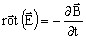# Physics reminder: conduction currents, induced currents, eddy currents

## Induced currents, eddy currents

When metallic conductors are inserted in a variable magnetic field or when they are in motion in a constant field, the result is the generation of electrical currents called induced currents or eddy currents in these conductors.

## Effects of induced currents

Induced currents have a negative effect in the core of transformers. This may be reduced by using thin laminations, which prevents the propagation of these currents.

They have a positive effect in induction heating or non-destructive control applications, to dampen the oscillations of moving parts, in rotor bars of induction machines, in the secondary circuit of transformers, etc.

## Induced currents, conduction currents

For the different cases analyzed, there are two possible current flows:

• the conduction current
• the induced current

These two possibilities are presented in the table below.

When the passing current

in the conductor …

we call this …
is imposed by an external source of current or voltage source

conduction current

(the conductor is supplied directly by a current or a voltage source)

is the result of the variation in time of the magnetic flux density, viz. of Faraday law:induced current :

(the conductor is supplied indirectly “by induction”)

## Example of a transformer

In a transformer we distinguish:

• conduction currents in the primary winding, copper or aluminum
• induced currents in the secondary winding, copper or aluminum
• eddy currents in the laminations of the magnetic circuit.